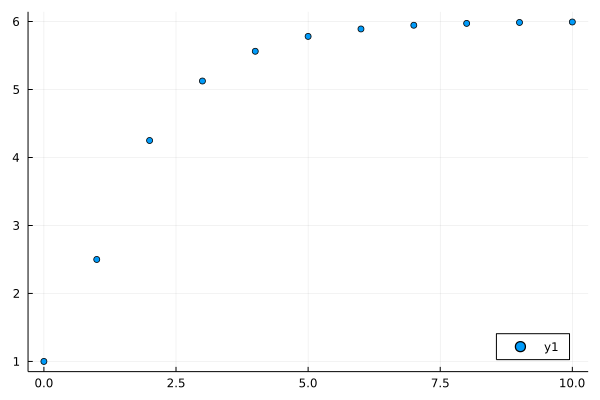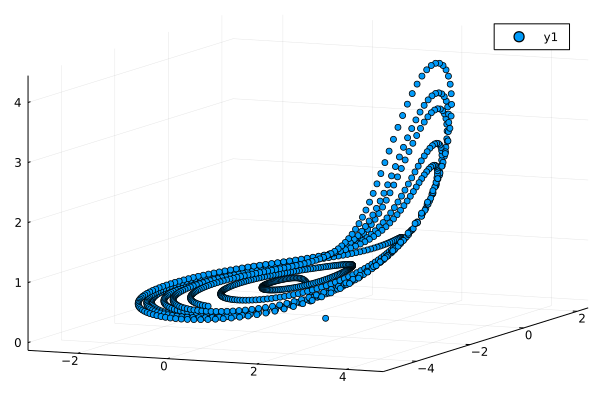# Fractional Differences Equations

There are also some solvers can be used to solve Fractional Differences Equations in FractionalDiffEq.jl.

Fractional Differences Equations with the form:

$$$\Delta^{\alpha}x(t)=f(t+\alpha,\ x(t+\alpha))$$$

With initial condition:

$$$x(0)=x0$$$

Let's see an example here, we have a fractional differences equation with initial condition:

$$$\Delta^{0.5}x(t)=0.5x(t+0.5)+1$$$$$$x(0)=1$$$

By using the PECEDifference solver in FractionalDiffEq.jl:

using FractionalDiffEq, Plots

fun(x) = 0.5*x+1
α=0.5; x0=1;
T=1; h=0.1
prob = FractionalDifferenceProblem(fun, α, x0)
sol=solve(prob, T, h, PECEDifference())
plot(sol, seriestype=:scatter, legend=:bottomright)

And plot the solution:## System of Fractional Difference Equations

Let's see if we have a system of fractional difference equations:

$$$\begin{cases} {_3^G\nabla}_k^\alpha x_1(k+1)=-0.05x_2(k)-0.05x_3(k)+0.01\tanh(x_2(k))\\ {_3^G\nabla}_k^\alpha x_2(k+1)=0.05x_1(k)+0.02x_2(k)+0.01\tanh(x_1(k))\\ {_3^G\nabla}_k^\alpha x_3(k+1)=0.1-0.2x_3(k)+0.05x_1(k)x_3(k)+0.01\tanh(x_3(k)) \end{cases}$$$

To solve this system of fractional difference equations, we only need to follow the procedure likewise:

using FractionalDiffEq, Plots

function sys!(du, u, p, t)
du = -0.05*u - 0.05*u + 0.01*tanh(u)
du = 0.05*u + 0.02*u + 0.01*tanh(u)
du = 0.1 - 0.2*u + 0.05*u*u + 0.01*tanh(u)
end
prob = FractionalDifferenceSystem(sys!, 0.98, [1, -1, 0])
result = solve(prob, 7, GL())

plot(result[1, :], result[2, :], result[3, :], seriestype=:scatter)• 1Yiheng Wei, Jinde Cao, Chuang Li, Yang Quan Chen: How to empower Grunwald–Letnikov fractional difference equations with available initial condition?